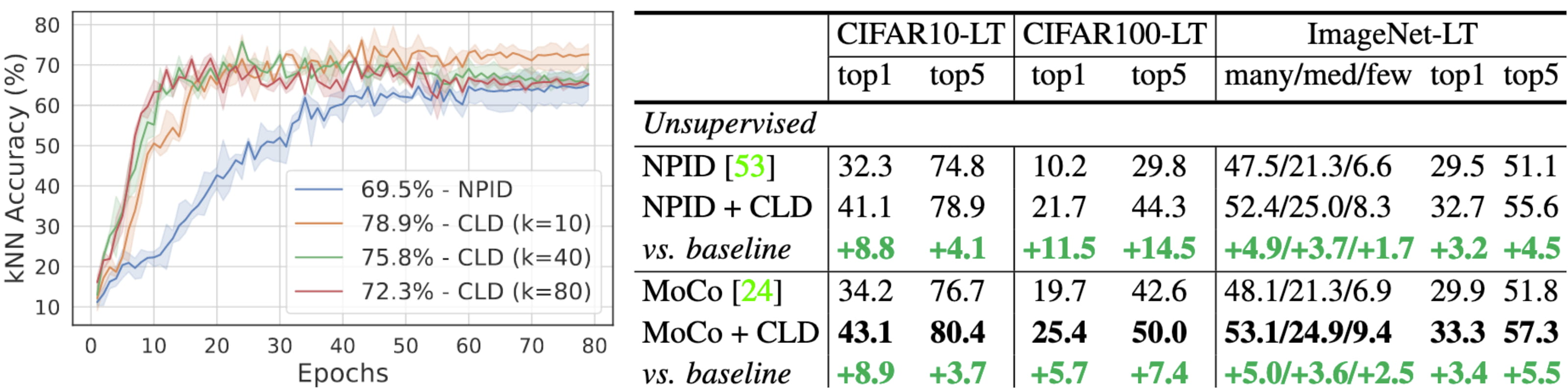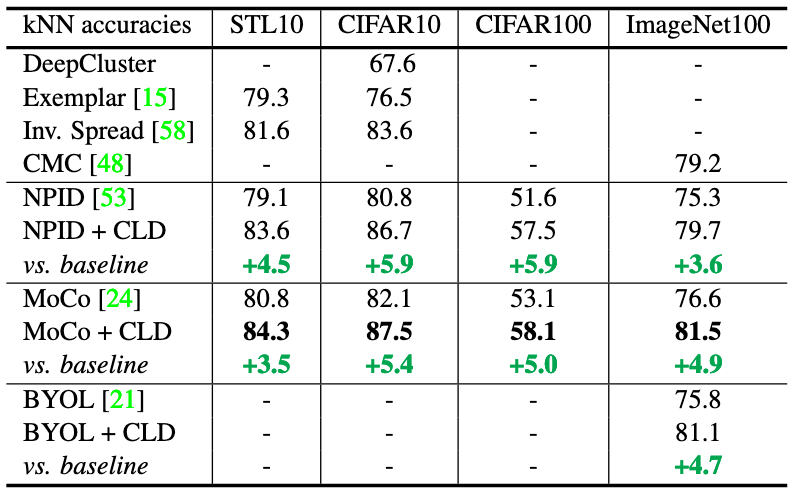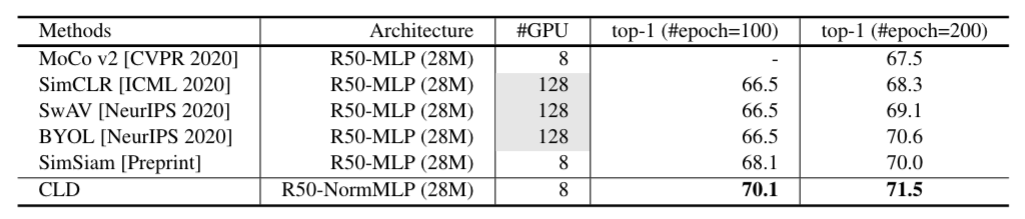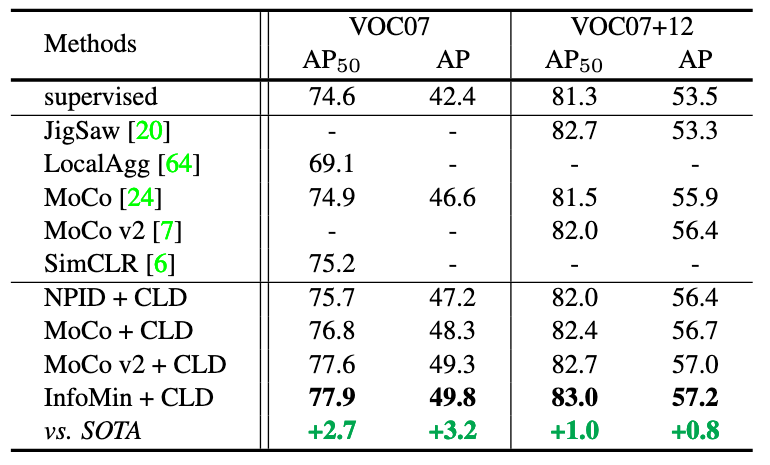# CLD: Unsupervised Feature Learning by Cross-Level Instance-Group Discrimination

## ABSTRACT

-----

Unsupervised feature learning has made great strideswith contrastive learning based on instance discrimination and invariant mapping, as benchmarked on curated class-balanced datasets. However, natural data could behighly correlated and long-tail distributed. Natural between-instance similarity conflicts with the presumed instance distinction, causing unstable training and poor performance.

Our idea is to discover and integrate between-instance similarity into contrastive learning, not directly by instance grouping, but by cross-level discrimination (CLD) between instances and local instance groups. While invariant mapping of each instance is imposed by attraction within its augmented views, between-instance similarity emerges from common repulsion against instance groups. Our batch-wise and cross-view comparisons also greatly improve the positive/negative sample ratio of contrastive learning and achieve better invariant mapping. To effect both grouping and discrimination objectives, we impose them on features separately derived from a shared representation. In addition, we propose normalized projection heads and unsupervised hyper-parameter tuning for the first time.

Our extensive experimentation demonstrates that CLD is a lean and powerful add-on to existing methods (e.g., NPID, MoCo, InfoMin, BYOL) on highly cor-related, long-tail, or balanced datasets. It not only achieves new state-of-the-art on self-supervision, semi-supervision, and transfer learning benchmarks, but also beats MoCo v2 and SimCLR on every reported performance attained with a much larger compute. CLD effectively extends unsupervised learning to natural data and brings it closer toreal-world applications.

## METHOD

-----

#### Method overview

Our goal is to learn representation $$f(x_i)$$ given image $$x$$ and its alternative view $$x'$$ from data augmentation. We fork two branches from $$f$$: fine-grained instance branch $$f_I$$ and coarse-grained group branch $$f_G$$. All the computation is mirrored and symmetrical with respect to different views of the same instance.
1) Instance Branch: We apply contrastive loss (two bottom $$C$$'s) between $$f_I(x_i)$$ and a global memory bank $$v_i$$, which holds the prototype for $$x_i$$, computed from the average feature of the augmented set of $$x_i$$.
2) Group Branch: We perform local clustering of $$f_G(x_i)$$ for a batch of instances to find $$k$$ centroids, $$\{M_1,\ldots,M_k\}$$, with instance $$i$$ assigned to centroid $$\Gamma(i)$$. Their counterparts in the alternative view are $$f_G(x_i')$$, $$M'$$, and $$\Gamma'$$.
3) Cross-Level Discrimination: We apply contrastive loss (two top $$C$$'s) between feature $$f_G(x_i)$$ and centroids $$M'$$ according to grouping $$\Gamma'$$, and vice versa for $$x_i'$$.
4) Two similar instances $$x_i$$ and $$x_j$$, which we don't know a priori, would be pushed apart by the instance-level contrastive loss but pulled closer by the cross-level contrastive loss, as they repel common negative groups. Forces from branches $$f_I$$ and $$f_G$$ act on their common feature basis $$f$$, organizing it into one that respects both instance grouping and instance discrimination.Fig 1. Method overview.

#### Normalized Projection Heads

Existing methods map the feature onto a unit hypersphere with a projection head and then normalization. NPID and MoCo use one FC layer as the linear projection head. MoCo v2, SimCLR, and BYOL adopt a multi-layer perceptron (MLP) head for large datasets, though it could hurt small datasets. We propose to normalize both the FC layer weights $$W$$ and the shared feature vector $$f$$ so that projecting $$f$$ onto $$W$$ simply calculates their cosine similarity. The final normalized $$d$$-dimensional feature $$N(x_i)$$ has $$t$$-th component:

$${N_t(x_i) =\frac{<W_t, f(x_i)>}{|W_t| \cdot | f(x_i)|}, \quad t=1,\ldots, d.}$$

#### Our work makes 4 major contributions.

1) We extend unsupervised feature learning to natural data with high cor-relation and long-tail distributions.

2) We propose cross-level discrimination between instances and local groups, to discover and integrate between-instance similarity into contrastive learning.

3) We also propose normalized projection heads and unsupervised hyper-parameter tuning.

4) Our experimentation demonstrates that adding CLD and normlized project heads to existing methods has an negligible model complexity overhead and yet delivers a significant boost.

## Results

-----Fig 2. CLD outperforms unsupervised baselines on high-correlation dataset Kichen-HC (left) and long-tailed datasets (right).Fig 3. Consistent improvements can be observed on small- and medium-scale benchmarks.Fig 4. Linear classifier top-1 accuracy (%) comparison of self-supervised learning on ImageNet. CLD obtains SOTA among all methods under 100-epoch and 200-epoch pre-training.Fig 5. Comparison with state-of-the-arts under 100 and 200 training epochs.Fig 6. Top-1 accuracy of semi-supervised learning (1% and 10% label fractions) on ImageNet.Fig 7. Transfer learning results on object detection.

-----

## CITATION

-----

@article{wang2020unsupervised,
title={Unsupervised Feature Learning by Cross-Level Instance-Group Discrimination},
author={Wang, Xudong and Liu, Ziwei and Yu, Stella X},
journal={arXiv preprint arXiv:2008.03813},
year={2020}
}